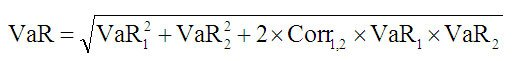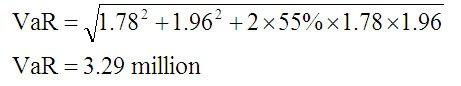# Value at Risk (VaR) of a Portfolio

Value-at- Risk (VaR) is a general measure of risk developed to equate risk across products and to aggregate risk on a portfolio basis. VaR is defined as the predicted worst-case loss with a specific confidence level (for example, 95%) over a period of time (for example, 1 day). For example, every afternoon, J.P. Morgan takes a snapshot of its global trading positions to estimate its DEaR (Daily-Earnings-at-Risk), which is a VaR measure defined as the 95% confidence worst-case loss over the next 24 hours due to adverse price moves.

This definition of VaR uses a 5% risk level: You would anticipate exceeding your VaR amount only 5% of the time (or, 95% of the time you expect to lose less than your VaR amount) over a 1-day horizon.

We will now learn how to calculate the VaR of one position and two positions by applying the concept of volatilities and correlations.

The following examples of how to calculate the risk of one and two positions illustrate the basic concept of parametric (delta) VaR estimation for linear instruments.

The general steps for calculating VaR are:

Step 1: Set VaR parameters: probability of loss and confidence level, time horizon, and base currency.

Step 2: Determine market value of each position, in base currency.

Step 3: Calculate VaR of individual positions, given market volatilities.

Step 4: Calculate portfolio VaR, given correlations between all variables.

VaR can be estimated as follows:

VaR = Market value X Price volatility

We will generally use the term volatility to express a multiple of standard deviation, depending on our chosen confidence level for VaR (i.e., confidence level multiple X standard deviation). Therefore, if we are using a VaR confidence level of 95%, volatility will refer to 1.65 X standard deviation.

## Risk of a Single Position

AlphaSquare are a USD-based firm with one asset: JPY 14 billion in cash. What is the 95% worst-case loss over a 1-day period?

You have the following information:

• The daily price volatility of the JPY/USD exchange rate is 1.78%, using a 95% confidence level. (Note: This implies that 1 standard deviation equals 1.78%/1.65 = 1.08%.)
• The JPY/USD exchange rate is 140.

### Solution

StepCalculationComment
1. Choose a probability of loss5%\=95% confidence worst-case loss
2. Measure value in USD$100 millionAssuming 140 JPY/USD 3. 1-day volatility JPY/USD (or 1.65 standard deviations)1.78%Data Set 4. Calculate Risk$100 million * 1.78 = $1.78Market value volatility This means that your 95% worst-case loss due to adverse movements in the JPY IUSD over 1 day would be$1.78 million (or, you have a 5% chance of losing $1.78 million or more overnight). Now let's view an example of two cash positions. To calculate the total risk of two or more positions, however, we need to include correlations. ## Risk of Two Cash Positions You now have two assets: JPY 1 billion + THB 4 billion. What is the risk over a 1-day period? ### Solution StepCalculationComment 1. Measure value in USD$200 million140 JPY/USD and 40 THB/USD
2. 1-month volatilityJPY/USD 1.78%
THB/USD 1.96%
Dataset
3. What are risks?/$On JPY$ 1.78 million
On THB $1.96 million Data Set 4. What is total risk?$ 1.78 + $1.96 m =$ 3.74 millionThis is incorrect you cannot just add the risks!

Simply adding risks together is incorrect: This would assume that both JPY and THB would move together in perfect lockstep. To calculate the total risk of two or more positions, we need to know correlations between all variables.

The data sets shows a correlation of 55% between JPY/USD and THB/USD.

Because the correlation is less than 1, we expect the total risk of our JPY and THB positions to be less than $3.74 million. Undiversified risk$3.74. million
Diversification benefit-$0.45 million Net risk$3.29 million

The diversification shows the difference between net portfolio risk and gross risk assuming perfect correlation (i.e., net portfolio risk minus gross risk).

We can calculate the risk of two linear positions using the following formula:In our example, the calculation will be as follows: